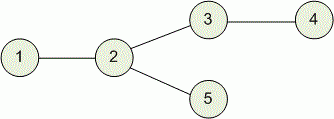Competitions

# Distance in Tree

A tree is a connected graph that doesn't contain any cycles.

The distance between two vertices of a tree is the length (in edges) of the shortest path between these vertices.

You are given a tree with n vertices and a positive number k. Find the number of distinct pairs of the vertices which have a distance of exactly k between them. Note that pairs (v, u) and (u, v) are considered to be the same pair.

#### Входные данные

The first line contains two integers n and k (1 ≤ n ≤ 50000, 1 ≤ k ≤ 500) - the number of vertices and the required distance between the vertices.

Next n - 1 lines describe the edges as ai bi (1 ≤ ai, bi ≤ n, ai ≠ bi), where ai and bi are the vertices connected by the i-th edge. All given edges are different.

#### Output

Print a single integer - the number of distinct pairs of the tree's vertices which have a distance of exactly k between them.Time limit 1 second
Memory limit 128 MiB
Input example #1
5 2
1 2
2 3
3 4
2 5

Output example #1
4

Source VK Cup 2012 Round 1, Problem D